/

### Derivative Pricing – Cost of Capital Pricing Model

1.            A simple way of incorporating in derivative pricing the possibility that investment markets might jump is to consider the limiting (and therefore typically unrealistic) case where the price of the underlying might jump to zero or infinity. This is the basis of the ‘cost of capital’ developed by M.H.D. Kemp and A.D. Smith, see e.g. Kemp (1997) or Kemp (2009).

2.            This model assumes that in any small instant the underlying may jump in price either infinitely upwards or down to zero.  An option writer is putting his or her capital at risk from such jumps as they are not hedgeable by investing merely in the underlying and risk-free assets.  It is reasonable to assume that the option writer will demand an excess return on the ‘risk capital’ that the option writer needs, to reflect this risk.  Of course, such risks can be hedged by buying suitable options, but this has merely transferred the jump risk to someone else.  Ultimately, someone must carry this risk.

3.            The model thus has two additional parameters to those applicable to the Black-Scholes formulae, namely: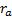= the rate of interest required on the cash the writer would need to hold to make good the loss that he would incur if there were an extreme downward jump in the price of the underlying  shares and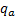= the enhanced income yield required on the shares the writer would need to hold to make good the loss that he would incur if there were an extreme upward movement in the price of the underlying shares

4.            The values under this model of European put options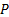and call options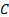are as follows, see Kemp (1997) for a derivation. They bear some resemblance to the formulae applicable in the more basic Black-Scholes world (and reproduce them in the special case where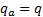and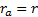).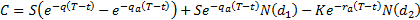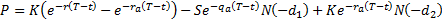where: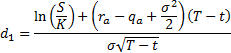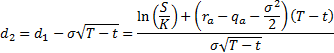5.            The Nematrian website includes functions for pricing European call and put options given the ‘cost of capital’ model, namely MnCCCall and MnCCPut respectively. The ‘CC’ here refers to the cost of capital model, to differentiate the pricing model from the basic Black-Scholes model, designated within the Nematrian web services toolkit by ‘BS’.